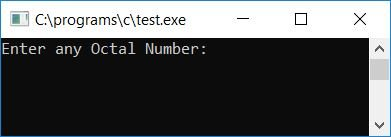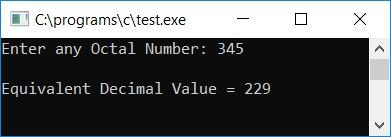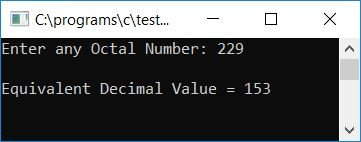# C Program to Convert Octal to Decimal

In this article, we will learn how to create a program in C that can convert any given octal number into its equivalent decimal number. At last, we will also take a look at a function-driven program that does the same job but uses a user-defined function named OctToDec().

But before going through the program. If you are not aware of

• Octal Number
• Decimal Number
• Octal to Decimal Conversion Process

then refer to the step-by-step process for octal to decimal conversion. Now let's move on to the program.

## Octal to decimal in C

To convert an octal number to a decimal number in C programming, you have to ask the user to enter the octal number first. Then convert it into a decimal number, and then display the equivalent value in decimal form as output.

```#include<stdio.h>
#include<conio.h>
#include<math.h>
int main()
{
int octnum, decnum=0, i=0, rem;
printf("Enter any Octal Number: ");
scanf("%d", &octnum);
while(octnum!=0)
{
rem = octnum%10;
decnum = decnum + (rem*pow(8,i));
i++;
octnum = octnum/10;
}
printf("\nEquivalent Decimal Value = %d", decnum);
getch();
return 0;
}```

Because the above program was written in the Code::Blocks IDE, you will receive the following output after a successful build and run. This is the first snapshot of the sample run:Now supply any octal number, say 345, as input and then press the ENTER key to see the output that shows you its equivalent decimal value as shown in the second snapshot given below:#### Program Explained

• Receive any octal number, say 345, in the octnum variable.
• Create a while loop that runs until the value of octnum becomes zero.
• Because the condition octnum!=0 or 345!=0 evaluates to true on the first run, program flow is directed inside the loop.
• And octnum%10 or 345%10 or 5 gets initialized to rem, and then decnum + (rem*pow(8,i)) (here, decnum and i were initialized with 0 at the start of the program) or 0 + (5*pow(8,0)) or 0 + (5*80) or 0 + 5*1 or 5 gets initialized to decnum.
• The value of i goes up by 1 and becomes 1, and the value of octnum/10, 345/10, or 34 is set to octnum.
• Now the program flow goes back to the condition of the while loop, and this time again octnum!=0 or 34!=0 evaluates to true. The program flow again goes back inside the loop.
• And octnum%10 or 34%10 or 4 gets initialized to rem.
• decnum is set to 37 by decnum + (rem*pow(8, i)) or 5 + (4*pow(8, 1)) or 5 + 4*81 or 5 + 32.
• The value of i is increased to 2, and the value of octnum/10, 34/10, or 3 is set to octnum.
• Now the program flow goes back to the condition of the while loop and the condition octnum!=0 or 3!=0 evaluates to true, and the program flow again goes back inside the loop
• And octnum%10 or 3%10 or 3 gets initialized to rem, and then decnum + (rem*pow(8, i)) or 37 + (3*pow(8, 2)) or 37 + 3*82 or 37 + 192 or 229 gets initialized to decnum.
• The value of i gets incremented and becomes 3, and octnum/10 or 3/10 or 0 gets initialized to octnum.
• Now the program flow goes back again to the condition of the loop, and this time octnum!=0 or 0!=0 evaluates to be false, therefore program flow exits from the while loop, and the value of decnum which is 229 gets printed as equivalent decimal value of given octal number 345 as output.
• Here we have used the function pow() of the math.h library, which takes two arguments, the first of which is responsible for the base and the second for the exponent. In 81, for example, the base is 8 and the exponent is 1.

### Octal to Decimal in C without the pow() Function

You can also create the program without using the pow() function of the math.h library. Here is the program without using any pre-defined functions:

```#include<stdio.h>
#include<conio.h>
int main()
{
int octnum, decnum=0, rem, mul=1;
printf("Enter any Octal Number: ");
scanf("%d", &octnum);
while(octnum!=0)
{
rem = octnum%10;
decnum = decnum + (rem*mul);
mul = mul*8;
octnum = octnum/10;
}
printf("\nEquivalent Decimal Value = %d", decnum);
getch();
return 0;
}```

Here is the final snapshot of the above program's sample run:### Octal to Decimal in C using a User-Defined Function

Let's create a function-driven program in which the function OctToDec() is created in such a way that it takes one argument as an octal number, converts it into its equivalent decimal value, and then returns it to the main() function, as shown in the program given below:

```#include<stdio.h>
#include<conio.h>
int OctToDec(int oct);
int main()
{
int octnum, decnum=0, rem, mul=1;
printf("Enter any Octal Number: ");
scanf("%d", &octnum);
decnum = OctToDec(octnum);
printf("\nEquivalent Decimal Value = %d", decnum);
getch();
return 0;
}
int OctToDec(int oct)
{
int rem, dec=0, mul=1;
while(oct!=0)
{
rem = oct%10;
dec = dec + (rem*mul);
mul = mul*8;
oct = oct/10;
}
return dec;
}```

The final snapshot of the above program's sample run is given below:#### The same program in different languages

C Quiz

« Previous Program Next Program »